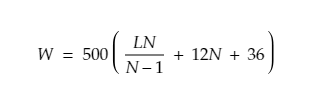• Instantly Determine Permit & Escort Costs
• Calculate Max Legal/Permitted Axle Weights
• Understand Equipment Limits

# Federal Bridge Formula Calculator | Bridge Law Formula

Federal Bridge Formula establishes the maximum weight any set of axles may legally carry on the Interstate roadways. The FBF is a mathematical formula used to determine the appropriate weight of loads based on axle configuration (number of axles, axle spacing, weight distribution). The formula is as follows:• W = the maximum weight in pounds that can be carried on a group of two or more axles to the nearest 500 pounds (230 kg).
• L = spacing in feet between the outer axles of any two or more consecutive axles.
• N = number of axles being considered.

This formula can look intimidating, because of that, Oversize.io has developed a calculator to determine whether your load meets FBF guidelines. (See Also: State Axle Weight Regulations)